# step by step带你HAN文本分类

**论文概述
**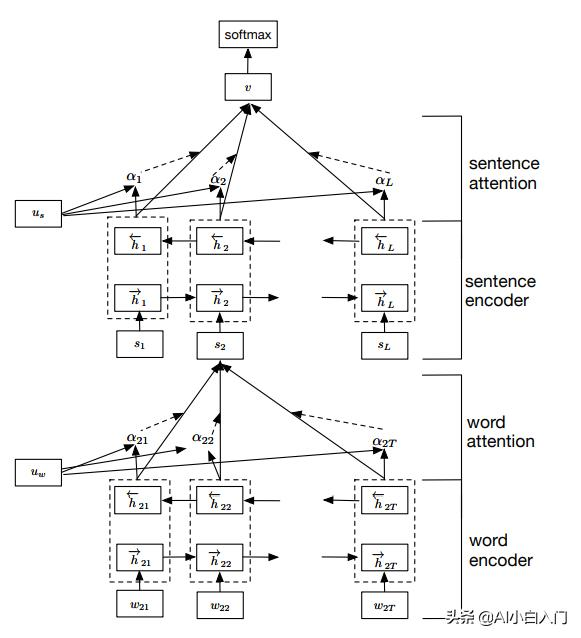• word encoder （BiGRU layer）
• word attention （Attention layer）
• sentence encoder （BiGRU layer）
• sentence attention （Attention layer）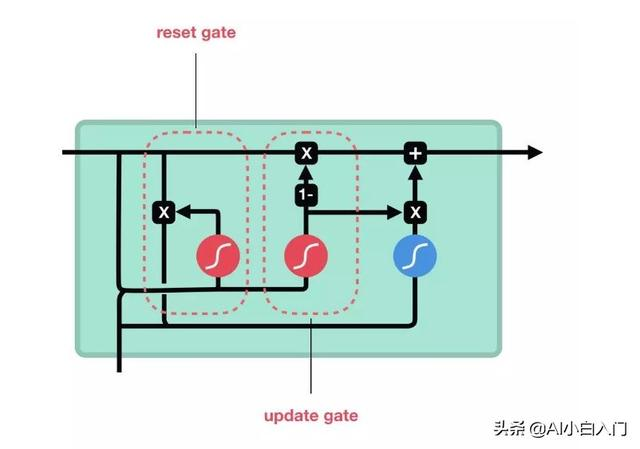GRU是RNN的一个变种，使用门机制来记录当前序列的状态。在GRU中有两种类型的门（gate）:reset gate和update gate。这两个门一起控制来决定当前状态有多少信息要更新。

reset gate是用于决定多少过去的信息被用于生成候选状态，如果Rt为0，表明忘记之前的所有状态：

update gate是用来决定有多少过去的信息被保留，以及多少新信息被加进来：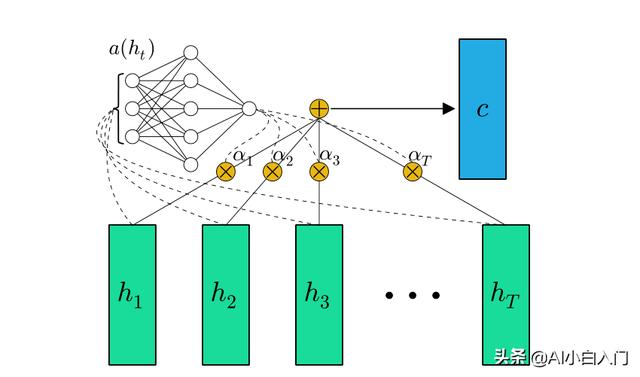#### 2、word attention layer

attention机制的目的就是要把一个句子中，对句子表达最重要的单词找出来，赋予一个更大的比重。

#### 6、模型效果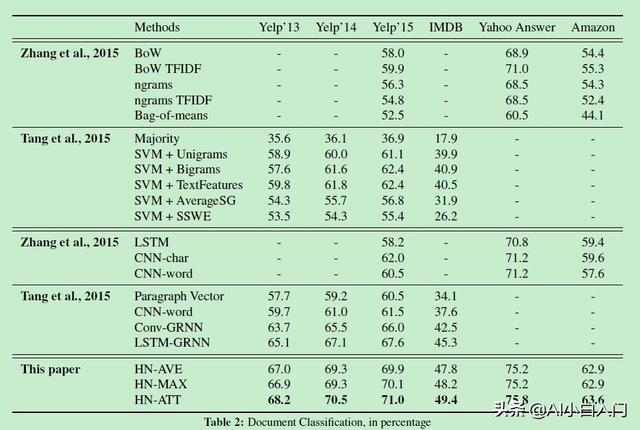## 代码实现

#### 定义模型

class HAN(object):
def __init__(self, max_sentence_num, max_sentence_length, num_classes, vocab_size,
embedding_size, learning_rate, decay_steps, decay_rate,
initializer=tf.random_normal_initializer(stddev=0.1)):
self.vocab_size = vocab_size
self.max_sentence_num = max_sentence_num
self.max_sentence_length = max_sentence_length
self.num_classes = num_classes
self.embedding_size = embedding_size
self.hidden_size = hidden_size
self.learning_rate = learning_rate
self.decay_rate = decay_rate
self.decay_steps = decay_steps
self.l2_lambda = l2_lambda
self.initializer = initializer
self.global_step = tf.Variable(0, trainable=False, name='global_step')
# placeholder
self.input_x = tf.placeholder(tf.int32, [None, max_sentence_num, max_sentence_length], name='input_x')
self.input_y = tf.placeholder(tf.int32, [None, num_classes], name='input_y')
self.dropout_keep_prob = tf.placeholder(tf.float32, name='dropout_keep_prob')
if not is_training:
return
word_embedding = self.word2vec()
sen_vec = self.sen2vec(word_embedding)
doc_vec = self.doc2vec(sen_vec)
self.logits = self.inference(doc_vec)
self.loss_val = self.loss(self.input_y, self.logits)
self.train_op = self.train()
self.prediction = tf.argmax(self.logits, axis=1, name='prediction')
self.pred_min = tf.reduce_min(self.prediction)
self.pred_max = tf.reduce_max(self.prediction)
self.pred_cnt = tf.bincount(tf.cast(self.prediction, dtype=tf.int32))
self.label_cnt = tf.bincount(tf.cast(tf.argmax(self.input_y, axis=1), dtype=tf.int32))
self.accuracy = self.accuracy(self.logits, self.input_y)

• END -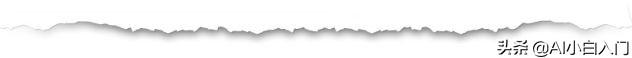The End©️2019 CSDN 皮肤主题: 数字20 设计师: CSDN官方博客